Function Repository Resource:

# TupperDecipher

Decrypt with Tupper’s self-referential formula

Contributed by: Aster Ctor
 ResourceFunction["TupperDecipher"][secret,p] decrypts integer secret using prime key p.

## Details and Options

Tupper’s self-referential formula is a formula that visually represents itself when graphed at a specific location in the xy-plane.
The formula was defined by Jeff Tupper and appears as an example in Tupper's 2001 SIGGRAPH paper on reliable two-dimensional computer graphing algorithms.
ResourceFunction["TupperDecipher"] decodes an integer k into an image according to this principle.
The following options can be used to control the output style:
 Mesh True Frame True FrameStyle Opacity FrameTicksStyle Opacity FrameTicks All
The option "Column" can be used to control the number of columns in the output.

## Examples

### Basic Examples (2)

Decrypt Tupper’s self-referential formula:

 In:=Out=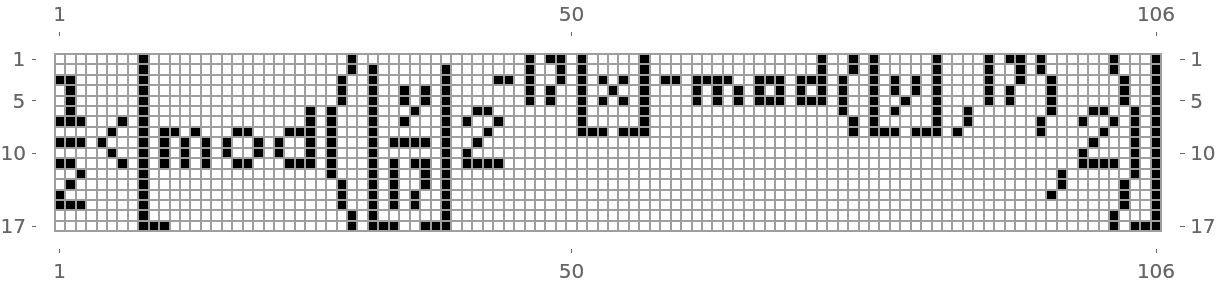Decrypt some other pattern:

 In:=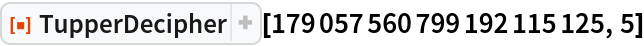Out=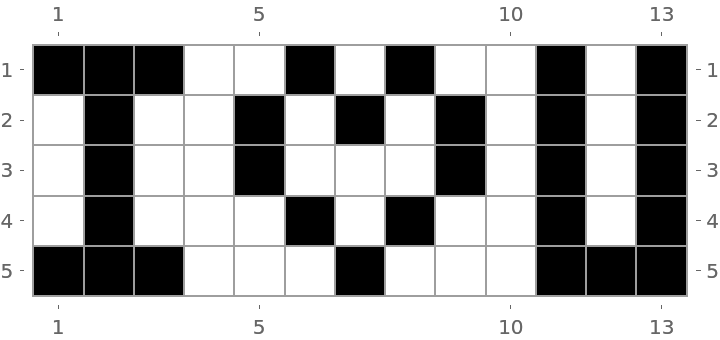### Options (1)

"Column" is a positive integer used to control the number of columns in the output:

 In:=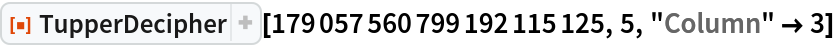Out=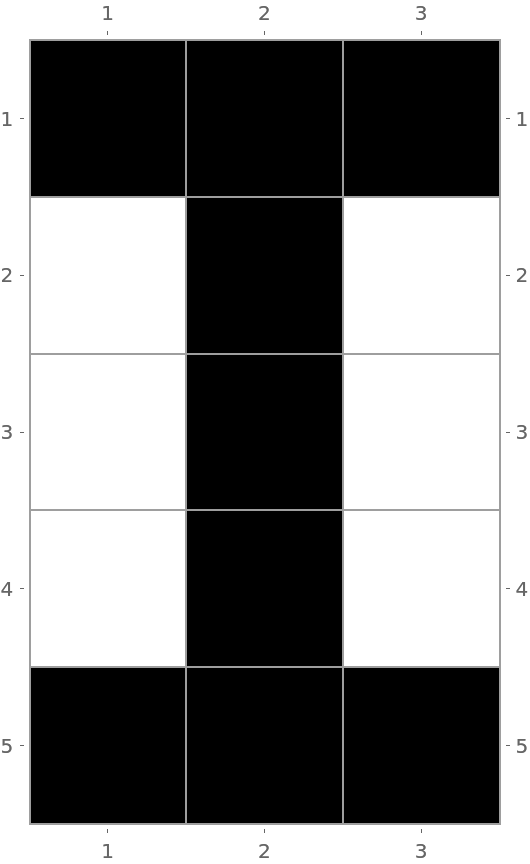### Possible Issues (4)

Here is a secret:

 In:=The correct key gives recognizable results:

 In:=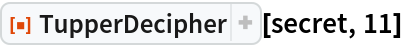Out=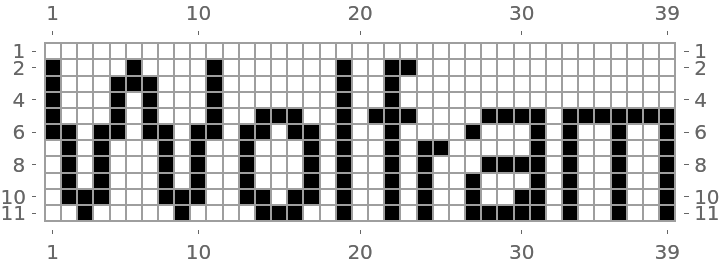Some keys cannot be used to decode secret, in which case a message is issued:

 In:=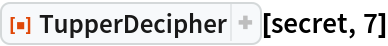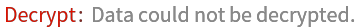Sometimes secret can be unlocked but it is unrecognizable:

 In:=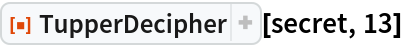Out=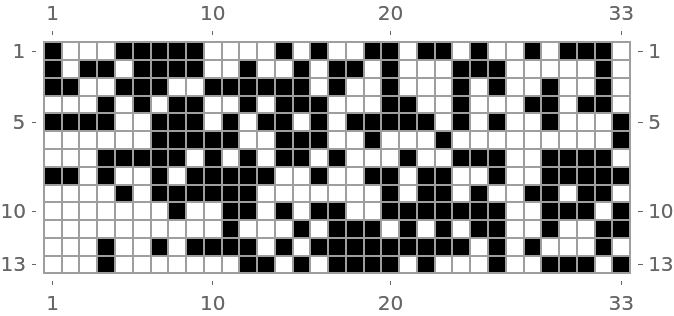## Publisher

Aster Ctor (MoeNet)

## Version History

• 2.0.0 – 30 December 2019
• 1.0.0 – 26 December 2019